# Earth’s Temperature Variation Emissions Boost Factor

The Temperature Variation Emissions Boost factor,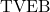, is defined by:

(1)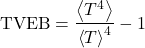is a factor that needs to be taken into account in a rigorous calculation of planetary temperature.

### Value of TVEB for the Moon

For convenience, I’ll use some data for the Moon from Nikolov & Zeller (2017) (though in general I do NOT endorse their work). N&Z assert that for the Moon, the average absorbed shortwave radiation is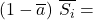294.0 W/m2, Global Mean Annual Temperature is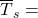197.35 K, and emissivity= 0.98.

From those number and my planetary temperature formula, I calculate that for the Moon,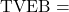2.49 and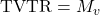= 0.732. So, if these numbers are accurate, thenis responsible for the Moon being 72 K colder than it would otherwise be.

The Moon experiences extreme temperature variations compared to Earth. For Earth, we expectto be much smaller. Indeed, it turns out to be much, much smaller.

### Value of TVEB for Earth

Based on the CERES SYN edition 4.1 Terra-Aqua dataset, I compute the typical value of Earth’sfor the period 2001-2021 to be= 0.0187. (Note, however, that calculations ofbased monthly data would yield a somewhat lower result, since diurnal variations and variation over the course of a month would not be accounted for in such a calculation.)

This corresponds to a Temperature Variation Temperature Reduction factor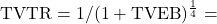0.99537.

Based on the same dataset, Earth’s average surface emissions for that period has been 398.87 W/m2. The corresponding mean “brightness temperature” for Earth’s surface (i.e, the temperature computed for an emissivity of 1) is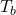= (398.87 W/m2 /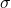)= 289.6 K / 16.5℃.

If the the Earth’s surface had a uniform temperature, thewould be 0. If everything else was held constant, that would lead to a brightness temperature of (289.6 K)/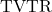= 290.95 K, which is 1.35℃ higher.

In conclusion, the factor(and) is responsible for reducing Earth’s global mean surface brightness temperature by around 1.35℃.

The factor(or) is in principle important in any rigorous calculation of equilibrium mean planetary surface temperature. However, these numbers indicate that, for Earth, the temperature-variation factorhas a relatively small impact.

This effect isn’t small for all planetary bodies. The Moon’s average surface temperature is around 90℃ colder than Earth’s, despite the Moon absorbing more sunlight per unit area than Earth does. Much of this enormous temperature reduction relative to Earth is due to the Moon’sfactor being quite large. (The Greenhouse effect is responsible for the remainder of the difference.)

### Contributions to TVEB

There are various ways of decomposinginto contributions from different types of temperature variation. Based on the CERES SYN data, here is one such decomposition (Decomposition A).1The values of variation over day and month were computed from CERES SYN 3-hour time resolution data for the year 2014, based on brightness temperature. The values for year, 20-year-period, longitude and latitude were computed from CERES SYN monthly average data, based on brightness temperature. The contribution due to emissivity distribution/variation was computed using CERES SYN 3-hour time resolution data for the year 2014, and comparing the results based on skin temperature to results based on brightness temperature.

Based on these results, the most important contributors to the overall value ofare, in descending order of importance, variations over latitude, variations over the annual cycle, and variations over longitude.

Different ways of decomposing the total result yield different attributions. I found that the largest difference in attribution occurs when the geographic calculations are done first, before time averaging. Doing that results in much less significance being attributed to temporal variations relative to geographic variations; in particular, the time-averaging contribution is reduced by over a factor of 10. However, since real datasets often involve some time averaging being done before one can do geographic averaging, the decomposition I’ve reported on may be of practical relevance.

### Uncertainty

Based on year-to-year variations in the calculatedvalue, the uncertainty inmay be around ± 0.0003. (I have not estimated any systematic uncertainty associated with the particular dataset that was analyzed.)